# NOTE: This set of www pages is not the set of www pages for the curent version of COMP101. The pages are from a previous version that, at the request of students, I have kept on line.

## CONTENTS

 1. Overview 1.1.Summary 2. Example problem - Decimal to binary conversion 2.1. Requirements 2.2. Analysis ` ` 2.3. Design 2.4. Implementation 2.5. Testing 3. Decimal to hexadecimal conversion 4. Alternative (recursive) implementation of Fibonacci Problem

The decimal to binary conversion example contains makes use of a default constructor, i.e. one that is not explicitly defined.

## 1. OVERVIEW

Recursion is a mechanism whereby repetition can be achieved using (at its simplest) a method that continues to call itself until some terminating value is reached, i.e. without using some specific repetition construct. The flow of control in recursion can be conceptualised as shown in the Activity Diagram given in Figure 1 (corresponding Nassi-Shneiderman chart in Figure 2). Not that, in Figure 1, the method call is a call to the same method as the calling method.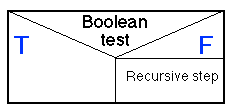Figure 2: NS chart indicating a recursive method

` `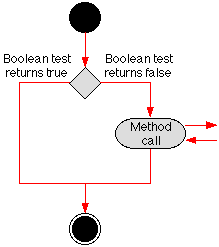Figure 1: Recursion Activity Diagram (1)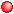Base case: The Boolean test at which the recursion ceases to "wind up" and starts to "unwind".Recursive step: A method call which causes the recursion to "repeat" on each successive iteration (unless the base case is reached).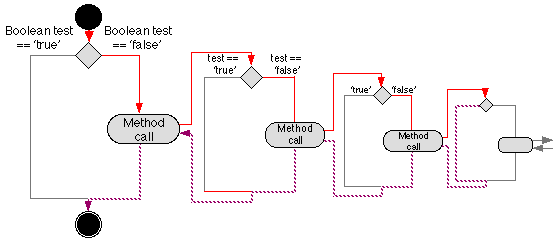Figure 3: Recursive flow of control

 The actual flow of control should therefore more accurately be described as shown in Figure 3. The Figure shows a recursive loop that iterates 4 times, i.e. the Boolean test is invoked on four occasions. The hrad (red) line marked with arrows shows the flow of control as the recursion winds up, i.e. until such a point is reached where the iterative process stops at which point the recursion is said to unwind --- the dashed (purple) line in the Figure. The light (Greyed out) processes and control flow lines show processes that are not invoked and lines that are not "exercised" in this case. The number of recursive iterations depends on the nature of the input to the Boolean test, i.e. anything between 0 (no iterations) and an infinite (never ending) loop. Consequently the approach to indicating the flow of control in recursion as shown in Figure 3 is not a good one --- we usually do not know in advance how many recursive iterations there will be. Consequently it is more convenient to indicate recursive flow of control as shown in Figure 4. Note in the figure that we have introduced some terminology specific to recursion: ` `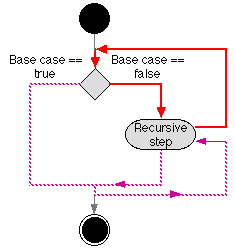Figure 4: Recursion Activity Digarms (2) Although Figure 4 is more succinct than Figure 3 the flow of control is not immediately obvious in all cases. It is clear that if the base case is reached immediately there is no recursive step and flow of control passes down the left hand path to the "sink". However, if the base case is reached after N iterations flow of control will initially again pass down the left hand branch but this time round to return to the recursive step. Flow of control will then loop for N iterations as the recursion unwinds (the purple line in Figure 4) until the recursion is fully unwound when flow of control will again pass on to "end".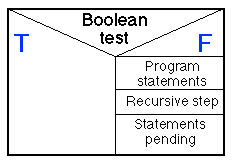Figure 6: NS chart depicting recursive method with statements pending ` ` This process is made even more confusing (at least to the novice) when the recursion includes statements to be executed before the recursive step, i.e. statement to be executed as the recursion winds up; and further statements pending to be executed as the recursion unwinds. The effect of this can best be visualised by returning to a AD of the form shown in Figure 3. This is presented in Figure 5. From this Figure we can clearly see that the statements pending will not be invoked until the recursion starts to unwind. For completeness, Figure 6 gives a NS chart depicting a recursive method with statements pending.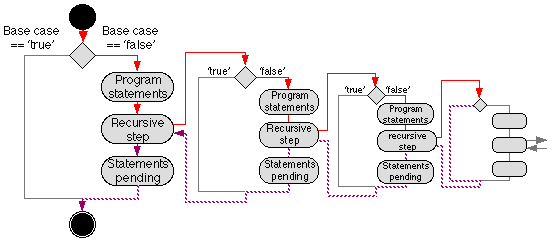Figure 5: Recursive Flow of Control with statements pending

### 1.1 SummaryRecursion is a process whereby a method calls itself so as to achieve repetitious behaviour.A recursive method comprises: A base case where the recursion stops and begins to unwind, and A recursive step where the method calls itself.We say that a recursive algorithm winds up until the base case is reached and then unwinds.
` `During the unwinding process further program statements "pending" may be called.

Note also that what has been described above is the simplest form of recursion over forms include "double recursion" (two recursive steps one pending), and "nested recursion" (one recursive step nested within another).

## 3. DECIMAL TO HEXADECIMAL CONVERSION

The recursive approach described above can be adapted to other types of integer conversion. For example decimal to hexadecimal conversion. The implementation for a suggested class Dec2Hex is presented in Table 4 (class digarm in Figure 12).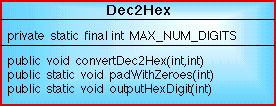Figure 12: Class diagarm for Dec2Hex conversion Class.

` `

Notes on code presented in Table 4The MAX_NUM_DIGITS constant is set to 3 becasuse the maximum number of digits to a 32 bit hexadecimal number is 4 (the count starts with digit 0).Note the use of the characters symbols 'A' through to and including 'F' to indicate the extra 6 digits available in the hexadecimal system.The Dec2Hex class presented here is designed to be used in conjunction with an application class very similar to the Dec2BinApp class introduced above (Table 2).
 ```// DECIMAL TO HEXADECIMAL COMVERSION // Frans Coenen // Thursday 1 July 1999 // The University of Liverpool, UK class Dec2Hex { // ------------------ FIELDS ------------------------ private static final int MAX_NUM_DIGITS = 3; // ------------------ METHODS ------------------------ /* ------ OUTPUT HEXADECIMAL NUMBER ------ */ /* Output a hexadecimal number with leading zeroes. */ public void convertDec2Hex(int number, int count) { final int HEX_BASE=16; // Base case if (number < HEX_BASE) { padWithZeros(count); outputHexDigit(number); } // Recursive step else { convertDec2Hex(number/HEX_BASE,count+1); outputHexDigit(number%HEX_BASE); } } /* ------ PAD WITH ZEROS ------ */ // Private class method to output a sequence of `0' characters to // pad the output with, where the hexadecimal number does not require // all the available digits for its encoding. private static void padWithZeros(int digitCount) { int loopParameter; int endValue = MAX_NUM_DIGITS-digitCount; // For loop, output a `0' on each iteration for(loopParameter=1;loopParameter< =endValue;loopParameter++) System.out.print(0x0); } /* ------ OUTPUT HEXADECIMAL DIGIT ------ */ /* Output a hexadecimal digit */ private static void outputHexDigit(int digit) { // Less than 10 not a problem. if (digit < 10) System.out.print(digit); // Else determine and iutput appropriate alphabetic literal else { switch (digit) { case 10: System.out.print('A'); break; case 11: System.out.print('B'); break; case 12: System.out.print('C'); break; case 13: System.out.print('D'); break; case 14: System.out.print('E'); break; default: System.out.print('F'); } } } } ```

Table 4: Implementation for dec2hex class

The application class to accompany the code presented in Table 4 is almost identical to that presented in table 2.

## ALTERNATIVE (RECURSIVE) IMPLEMENTATION OF FIBONACCI PROBLEM

In an earlier part of this "Introduction to Programming in Java" module we designed and implemented a program to output the Fibonacci sequence, using a for loop construct, up to a number of terms specified by the user (maximum of 45). An alternative approach to this problem is to use a recursive approach as shown in Table 5 and the applications class in Table 6. The class comprises a single method which calculates and returns the nth term in the Fibonacci sequence (unlike the previousn example method which output the entire sequence). The calculation is carried out using a double recursive technique to calculate pairs of terms that make up the next term. This approach nicely illustrates the use of this technique, however it is extremely inefficient.

 ```// FIBONACCI CLASS (VERSION 2) // Frans Coenen // Wednesday 23 June 1999 // The University of Liverpool, UK class FibonacciVer2 { // ------------------ METHODS --------------------- /* Calculate and output Fibonacci sequence */ public int calcFibonacciSeq(int numberOfTerms) { switch (numberOfTerms) { case 1: return(0); case 2: return(1); default: return(calcFibonacciSeq(numberOfTerms-1) + calcFibonacciSeq(numberOfTerms-2)); } } } ```

Table 5: Implementation for FibonacciVer2 class

 ```// FIBONACCI APPLICATION CLASS (VERSION 2) // FIBONACCI APPLICATION CLASS // Frans Coenen // Wednesday 23 June 1999 // Revised: Friday 26 August 2005 // The University of Liverpool, UK import java.util.*; class FibonacciVer2App { // ------------------- FIELDS ------------------------ // Create Scanner class instance public static Scanner keyboardInput = new Scanner(System.in); // ------------------ METHODDS ----------------------- /* Main method */ public static void main(String[] args) { int numTerms; final int MAX_TERMS = 45; final int MIN_TERMS = 0; // Input number of terms System.out.println("Input Number of terms (int " + MIN_TERMS + ".." + MAX_TERMS + "): "); do { numTerms = keyboardInput.nextInt(); } while (numTerms < MIN_TERMS || numTerms > MAX_TERMS); // Create instance of class Fibonacci FibonacciVer2 newFibonacci = new FibonacciVer2(); // Calculate and output result System.out.println("fib(" + numTerms + ") = " + newFibonacci.calcFibonacciSeq(numTerms)); } } ```

Table 6: Implementation for FibonacciVer2App class

Created and maintained by Frans Coenen. Last updated 10 February 2015